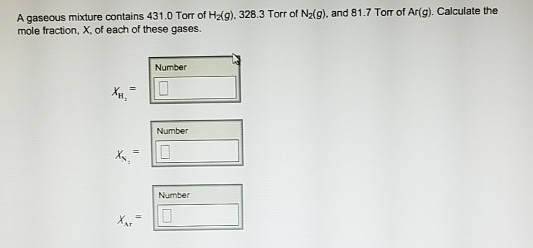# Problem: A gaseous mixture contains 431.0 Torr of H2(g), 328.3 Torr of N2(g), and 81.7 Torr of Ar(g). Calculate the mole fraction, X, of each of these gases.

###### FREE Expert Solution
79% (325 ratings)###### Problem Details

A gaseous mixture contains 431.0 Torr of H2(g), 328.3 Torr of N2(g), and 81.7 Torr of Ar(g). Calculate the mole fraction, X, of each of these gases.